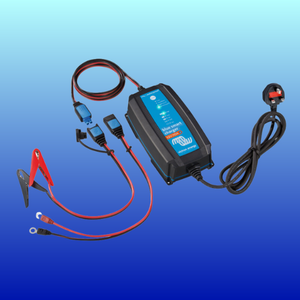# How much current is your 12v DC charger pulling from the mains?

We often get asked about the current draw of a 12v DC mains charger on the AC mains side. We have got a sum that will give you an approximate value of how many Amps a 12V charger will draw from the mains.

If you take the DC output Voltage of the charger, multiply it the by the number of Amps that it outputs and then divide it by the AC mains Voltage (in the UK it is around 230v) this will give you the number of Amps the charger will draw from the mains.So if you had a 12V, 10A mains charger, this would be the sum:

12 (Volts) x 10 (Amps) ÷ 230 (Volts) = 0.52 (Amps from the mains).

This is an approximation only, and if you want a more accurate figure then you could use the maximum DC charging voltage of around 14V and factor in the efficiency of the charger, which would give you a slightly higher current draw on the AC mains side.

Note that you can use the same equation for a 24V or 48V battery system.

I hope this sum will be useful to you and as always, if you have any questions regarding this article or anything else regarding your electrical system, contact us at sales@12voltplanet.co.uk and we will be happy to help.

 Share Tweet

Jeremy Burgum
08 September 2023  |  11:10

Given a charger produces more than 12V, I would use the highest voltage that the charger produces (e.g. 14.6V). The charger is only about 90% efficient so Id multiply the watts by 1.1.

In your example, 14.6 x 10 x 1.1 / 230 = 0.7A